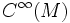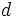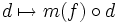Lie algebra of first-order differential operators

This article defines a basic construct that makes sense on any differential manifold
View a complete list of basic constructs on differential manifolds
This article gives a global construction for a differential manifold. There exists a sheaf analog of it, that associates a similar construct to every open subset. This sheaf analog is termed: sheaf of first-order differential operators

Definition

Let$M$ be a differential manifold. Let$C^\infty(M)$ be the algebra of infinitely differentiable functions on$M$. The Lie algebra of first-order differential operators is defined as follows:

• As a set, it is the set of all maps from$C^\infty(M)$ to$C^\infty(M)$, that can be expressed as the sum of a derivation, and pointwise multiplication by a function. The derivation can be thought of as the pure first-order part, and the scalar multiplication can be thought of as the zeroth
• The$\R$-vector space structure is by pointwise addition and scalar multiplication.
• There is a natural$C^\infty(M)$-bimodule structure, by composition. In other words,$f \in C^\infty(M)$ acts on a first-order differential operator$d$ by:$d \mapsto m(f) \circ d$

where$m(f)$ denotes multiplication by$f$. Similarly, the right action is given by:$d \mapsto d \circ m(f)$